# Understanding Equivalent Fractions

Some fractions seem to be different but are the same in value. For example, 1/2, 2/4, 3/6, 4/8…and so on.

Such fractions when reduced to simplest form become the same fraction.

2/4 = 3/6 = 4/8…= 1/2

Such fractions are called equivalent fractions.

Equivalent fraction of a given fraction is found by multiplying or dividing its numerator and denominator by the same whole number.

For example, if we multiply the numerator and denominator of 2/3 by 4 we get

2/3 = (2 × 4) / (3 × 4) = 8/12 which is an equivalent fraction of 2/3.

Similarly, if we divide the numerator and denominator of 12/18 by 6 we get

12/18 = (12 ÷ 6) / (18 ÷ 6) = 2/3. So 12/18 is an equivalent fraction of 2/3 as well.

The pie below is cut into 10 equal slices. Shade 1/5 of this pie### Solution

Step 1:

The denominator of 1/5 is 5, so the pie can be divided into 5 equal parts of 2 slices each. (Because 10 ÷ 5 = 2)

Step 2:

We shade one of these 5 equal parts to get the following pie.Step 3:

We shaded 1 part out of 5. It does not matter how we choose the 1 part to shade.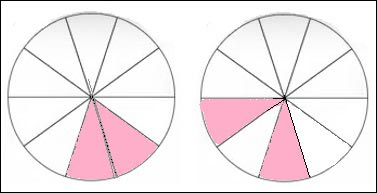The strip below is cut into 6 equal bars. Shade 2/3 of this strip.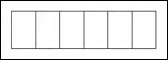### Solution

Step 1:

The denominator of 2/3 is 3, so the strip can be divided into 3 equal parts of 2 bars each. (Because 6 ÷ 3 = 2)

Step 2:

We shaded 2 parts out of 3.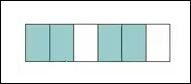Step 3:

It does not matter how we choose the 2 parts to shade.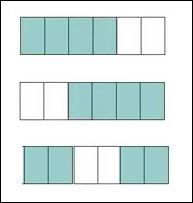2/3 × 2/2 = 4/6

2/3 × 3/3 = 6/9

2/3 × 4/4 = 8/12

2/3, 4/6, 6/9…are equivalent fractions

The pie below is cut into 10 equal slices. Shade 2/5 of this pie?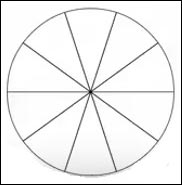### Solution

Step 1:

The given pie is cut into 10 equal slices.

The denominator of 2/5 is 5, so the pie can be divided into 5 equal parts of 2 slices each. (Because 10 ÷ 5 = 2). 2 parts means 4 slices.

Step 2:

So shading 4 slices or 2/5 of the pie, we get the following.2/5 and 4/10 are equivalent fractions